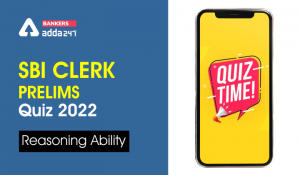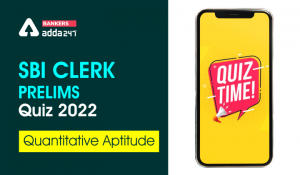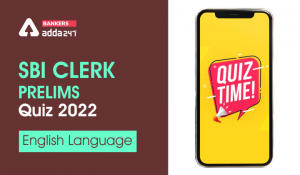Latest Banking jobs   »   Reasoning Ability Quiz For SBI Clerk...

# Reasoning Ability Quiz For SBI Clerk Prelims 2022- 2nd May

Directions (1-4): In the following questions assuming the given statement to be true, find which of the conclusion(s) among given conclusions is/are definitely true and then give your answers accordingly.

Q1. Statements: C > F, A < B ≤ E = G, F ≥ D = A
Conclusions:
I. C ≥ A
II. G > F
(a) Only I is true
(b) Only II is true
(c) Either I or II is true
(d) Neither I nor II is true
(e) Both I and II are true

Q2. Statements: R ≤ S = T, P = Q < R, T > U ≥ V
Conclusions:
I. P < T
II. S > V
(a) Only I is true
(b) Only II is true
(c) Either I or II is true
(d) Neither I nor II is true
(e) Both I and II are true

Q3. Statements: A = K ≥ L, J ≤ I < O, L > P = J
Conclusions:
I. O ≥ L
II. A > J
(a) Only I is true
(b) Only II is true
(c) Either I or II is true
(d) Neither I nor II is true
(e) Both I and II are true

Q4. Statements: R ≥ F > K, D > Q = R, K ≤ M < S
Conclusions:
I. Q > F
II. Q = F
(a) Only I is true
(b) Only II is true
(c) Either I or II is true
(d) Neither I nor II is true
(e) Both I and II are true

Directions (5-8): In the following questions assuming the given statement to be true, find which of the conclusion(s) among given conclusions is/are definitely true and then give your answers accordingly.

Q5. Statements: A = G > N ≥ W, V < B ≤ N, W > S = U
Conclusions:
I. A > B
II. N ≥ U
(a) Only I is true
(b) Only II is true
(c) Either I or II is true
(d) Neither I nor II is true
(e) Both I and II are true

Q6. Statements: S ≥ P = H < G, P < Y ≤ J = X, Y ≥ F = Z
Conclusions:
I. J > S
II. X > Z
(a) Only I is true
(b) Only II is true
(c) Either I or II is true
(d) Neither I nor II is true
(e) Both I and II are true

Q7. Statements: T > Y ≤ U = I > O, E = O > L = K, S = I > X ≤ C
Conclusions:
I. U > K
II. T > S
(a) Only I is true
(b) Only II is true
(c) Either I or II is true
(d) Neither I nor II is true
(e) Both I and II are true

Q8. Statements: O > E ≥ X < B > L, F = W > E ≤ G, S = X > Q
Conclusions:
I. F > O
II. O > Q
(a) Only I is true
(b) Only II is true
(c) Either I or II is true
(d) Neither I nor II is true
(e) Both I and II are true

Directions (9-11): In the following questions assuming the given statement to be true, find which of the conclusion(s) among given conclusions is/are definitely true and then give your answers accordingly.

Q9. Statements: J = E ≥ W = N, M < J ≤ H = Q, M > T = Y ≤ B
Conclusions:
I. Q > Y
II. J = B
(a) Only I is true
(b) Only II is true
(c) Either I or II is true
(d) Neither I nor II is true
(e) Both I and II are true

Q10. Statements: W < F = H > T ≥ O, E = K ≤ O ≤ M, I = C > E
Conclusions:
I. F > E
II. K < I
(a) Only I is true
(b) Only II is true
(c) Either I or II is true
(d) Neither I nor II is true
(e) Both I and II are true

Q11. Statements: U ≥ W ≥ B < N, W = X > S > Q, Y = R ≤ X > I
Conclusions:
I. U > S
II. S ≥ Y
(a) Only I is true
(b) Only II is true
(c) Either I or II is true
(d) Neither I nor II is true
(e) Both I and II are true

Directions (12-15): In the following questions assuming the given statement to be true, find which of the conclusion(s) among given conclusions is/are definitely true and then give your answers accordingly.

Q12. Statements: W ≤ F < G = B, Z ≥ S = M ≥ G, B < L = P, L ≤ S = E < C
Conclusions:
I. F < E
II. S ≤ B
(a) Only I is true
(b) Only II is true
(c) Either I or II is true
(d) Neither I nor II is true
(e) Both I and II are true

Q13. Statements: I > J ≥ M = B, P < S = R ≥ M, V > W = M ≥ C, R > D ≥ N = A
Conclusions:
I. N > W
II. J ≤ D
(a) Only I is true
(b) Only II is true
(c) Either I or II is true
(d) Neither I nor II is true
(e) Both I and II are true

Q14. Statements: O ≥ K ≥ M = W, Z = Q ≤ S ≤ W, V ≥ O = R < P, S = L > D ≥ E
Conclusions:
I. L < R
II. R = S
(a) Only I is true
(b) Only II is true
(c) Either I or II is true
(d) Neither I nor II is true
(e) Both I and II are true

Q15. Statements: K ≤ O = I, P < L = M ≤ K, I > J ≥ N ≥ B
Conclusions:
I. O ≥ L
II. K > J
(a) Only I is true
(b) Only II is true
(c) Either I or II is true
(d) Neither I nor II is true
(e) Both I and II are true

Solutions

S1. Ans. (d)
Sol.
I. C ≥ A – False
II. G > F –False

S2. Ans. (e)
Sol.
I. P < T – True
II. S > V -True

S3. Ans. (b)
Sol.
I. O ≥ L – False
II. A > J – True

S4. Ans. (c)
Sol.
I. Q > F – False
II. Q = F– False

S5. Ans. (a)
Sol.
I. A > B – True
II. N ≥ U – False

S6. Ans. (d)
Sol.
I. J > S – False
II. X > Z– False

S7. Ans. (a)
Sol.
I. U > K – True
II. T > S – False

S8. Ans. (b)
Sol.
I. F > O – False
II. O > Q – True

S9. Ans. (a)
Sol.
I. Q > Y – True
II. J = B – False

S10. Ans. (e)
Sol.
I. F > E – True
II. K < I – True

S11. Ans. (a)
Sol.
I. U > S – True
II. S ≥ Y – False

S12. Ans. (a)
Sol.
I. F < E – True
II. S ≤ B – False

S13. Ans. (d)
Sol.
I. N > W – False
II. J ≤ D – False

S14. Ans. (c)
Sol.
I. L < R – False
II. R = S – False

S15. Ans. (a)
Sol.
I. O ≥ L – True
II. K > J – False#### Congratulations!

Incorrect details? Fill the form again here

•Reasoning Ability Quiz For SBI Clerk Pre...
•Quantitative Aptitude Quiz For SBI Clerk...
•English Quizzes For SBI Clerk Prelims 20...
•Reasoning Ability Quiz For SBI Clerk Pre...
•English Quizzes For SBI Clerk Prelims 20...
•Reasoning Ability Quiz For SBI Clerk Pre...